# The three types of matrix row operations

### The three types of matrix row operations

In this section we will learn about matrix row operations. There are three types of matrix row operations: interchanging 2 rows, multiplying a row, and adding/subtracting a row with another. Each notation for this is different, and is displayed in the note section. First, we will look at questions which involve all three types of matrix row operations. Then we will look at more slightly advanced questions that involve using 2 matrix row operations.

#### Lessons

The first type of matrix row operation is that we are allowed to switch any rows you want. For example,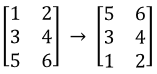We interchanged the row 1 and row 3. Another way of saying this is $R_1\leftrightarrow R_3$.

The second type of matrix row operation is that we can multiply a row by a non-zero constant. For example,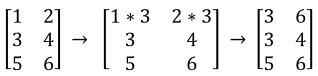We multiplied each entry in row 1 by 3. Another way of saying this is $3R_1\leftrightarrow R_1$.

The third type of matrix row operation is that we are allowed to add and subtract a row with another. For example,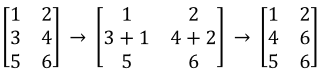Row 2 is the sum of row 2 and 1. Another way of saying this is $R_1+R_2$$R_2$.
• Introduction
Matrix row operations overview:

• 1.
One matrix row operation
You are given the following matrix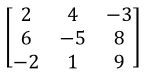Perform the following matrix operations:
a)
$R_1\leftrightarrow R_2$

b)
$R_2\leftrightarrow R_3$

c)
$-4R_3$$R_3$

d)
$3R_2$$R_2$

e)
$R_1+R_2$$R_2$

f)
$R_1+R_3$$R_3$

• 2.
More than one matrix row operation
You are given the following matrix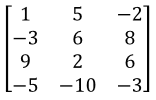Perform the following matrix operations:
a)
$3R_1+2R_4$$R_4$

b)
$2R_2-R_1$$R_2$

c)
$-2R_3+R_1$$R_1$

d)
$-2R_2+2R_3$$R_3$